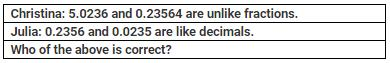Courses

# Test : Decimals

## 10 Questions MCQ Test Mathematics for Class 5 (V) - CBSE and NCERT Curriculum | Test : Decimals

Description
This mock test of Test : Decimals for Class 5 helps you for every Class 5 entrance exam. This contains 10 Multiple Choice Questions for Class 5 Test : Decimals (mcq) to study with solutions a complete question bank. The solved questions answers in this Test : Decimals quiz give you a good mix of easy questions and tough questions. Class 5 students definitely take this Test : Decimals exercise for a better result in the exam. You can find other Test : Decimals extra questions, long questions & short questions for Class 5 on EduRev as well by searching above.
QUESTION: 1

Solution:
QUESTION: 2

Solution:
QUESTION: 3

### X is a decimal which has three decimal places. If the decimal is converted fractional form, what will be the denominator for the resulting fraction?

Solution:
QUESTION: 4Solution:
QUESTION: 5

Which one of the following is the correct expanded form for the decimal 40.0235?

Solution:
QUESTION: 6

A and B are two decimals. When twice of A is subtracted from B we get 543.3202. If value of A is 548.355, find 2(A+B).

Solution:
QUESTION: 7

Jack selected two decimals 35.41 and 4.5 and multiplied them .The Product is 8.1443. After a while he realized that he mistakenly multiplied 35.41 by other decimal instead of 4.5. Find the other decimal.

Solution:
QUESTION: 8

X is 1459.55 more than Y and 542.31 less than Z. If X + Y = 1545.55. Find the value of X + Y + Z.

Solution:
QUESTION: 9

There are 4562 wheat bags in a godown. If each bag contains 45.6 kg of wheat, find the total amount of wheat in the godown.

Solution:
QUESTION: 10

Find the number which is 4.2456 less than the product of 45.21 and 9.76.

Solution: